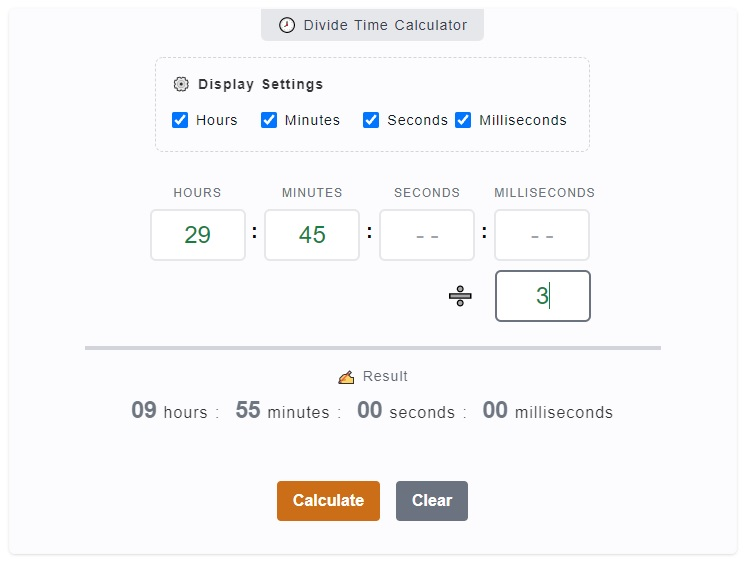# Divide Time Calculator

The Divide Time Calculator is used to get the quotient when a time is divided by a whole number. To put that on a practical example, the purpose of this calculator is to answer questions like this, what is `8 hours and 30 minutes` divided by `3`? You can enter these values on the calculator and you'll get the result.

🕗 Divide Time Calculator

:
:
:

### ✍ Result

00 hours : 00 minutes
00 seconds : 00 milliseconds

## How to Use the Divide Time Calculator

Doing some division of time when it's just simple whole numbers is really not that hard. Take for example, you want to divide 10 hours by 2, which you can just do the simple math and you'll get 5 hours.

However, if you are dealing now with complex numbers, it could be a bit difficult. Diving 29 hours and 45 minutes by 3, it could be challenging for some. That's why this calculator is built, to give you the answer right away.1. Step 1

The first thing you might want to do is to check whether you need all hours, minutes, seconds and milliseconds input fields. You can omit the one's you don't need by just unchecking the corresponding checkbox on the `⚙️ Display Settings`.

2. Step 2

Then, we proceed to the actual entering of time. On the calculator, there are input fields marked with hours, minutes, seconds and milliseconds. Enter your base time you want to divide on these fields. In mathematical division, it's called dividend. For example, enter 29 hours, 45 minutes, 50 seconds and 50 milliseconds.

3. Step 3

Following our example time entry above (`29 hours, 45 minutes, 50 seconds and 50 milliseconds`). We can now enter the divisor which is the input field beside the divide (➗) sign. It is the number we want to divide our time entry with. Let's add `3` on this field.

4. Step 4

After entering both the time (dividend) and (divisor), you can now click on the `Calculate` button to initialize the computation. The result (quotient) will be displayed at the bottom part of the calculator labeled with `✍ Result.`

## Divide Time Calculator Inputs and Outputs

The purpose of the calculator is to give you the result when you divide a time (HH:MM:SS) by a whole number. You can just check around the calculator and you'll be able to use it correctly. However, mentioning the inputs and outputs of this calculator would provide a better information.

### Display Settings

The display settings are added on the calculator for you to personalize your inputs. These are in the form of checkboxes, which is checked by default. It includes the `Hours`, `Minutes`, `Seconds` and `Milliseconds`. You can actually uncheck the one's you don't need and it will be hidden on the display.

### Inputs

There are two (input) groups on the calculator. The first one is the dividend which represents your time entry. Then, the other one is the divisor which is a number used to multiply your time with.

### Outputs

The output or the result of the calculation is being displayed right below the input fields which is labeled with `✍ Result`. This serves as the output or quotient when dividing time and a number.

### Calculate and Clear Buttons

Make sure to click on the `Calculate` button every time you have finished entering your inputs. You can also clear them by just clicking the `Clear` button.

## Video Explanation

Here's a video tutorial on how to use the Divide Time Calculator.